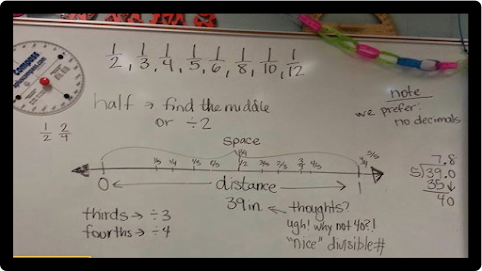Posts

Fraction Approximation - Visualizing fractions on a number line in the classroomI would like to tell you a little (maybe a long) story about how we do this in our classroom . I started out the lesson  by  drawing a random line on the board ( I really didn't measure it until we started the conversation). As   we chatted, we talked about how much "space" the line took up,  and the students decided that I needed to call that " distance ." Then, we   went on to talk about how we could choose where to mark our fractions on a number line.  For example, the   students told me that   we either needed to "find the  middle" or divide the line in half to find where half should be placed. At this point, I pulled out a tape   measure and measured the line 39 inches (inside,  I was ecstatic that it was only going to be divisible by 3!) You may notice what I am telling you now is also written on the whiteboard in the last picture.   I